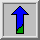# Blending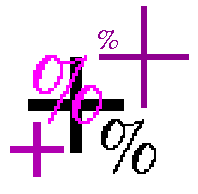Common Names: Blend, Merge

## Brief Description

This operator forms a blend of two input images of the same size. Similar to pixel addition, the value of each pixel in the output image is a linear combination of the corresponding pixel values in the input images. The coefficients of the linear combination are user-specified and they define the ratio by which to scale each image before combining them. These proportions are applied such that the output pixel values do not exceed the maximum pixel value.

## How It Works

The resulting image is calculated using the formula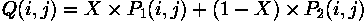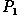and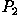are the two input images. In some applicationscan also be a constant, thus allowing a constant offset value to be added to a single image.

X is the blending ratio which determines the influence of each input image in the output. X can either be a constant factor for all pixels in the image or can be determined for each pixel separately using a mask. The size of the mask must then be identical with the size of the images.

Some implementations only support graylevel images. If multi-spectral images are supported the calculation is done for each band separately.

## Guidelines for Use

Image blending is used for similar applications as image addition with the difference that we don't have to worry whether the values of the output image exceed the allowed maximum. In most cases the operator is a part of some more complicated process. As an example we use image blending to overlay the output of an edge detector on top of the original image, (compare with the results achieved with image addition).

The imageshows a simple flat dark object against a light background. Applying the Canny edge detector to this image we obtain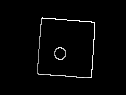We get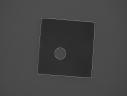if we apply the blending operator with X = 0.5, where the original image isand the edge image is. The result clearly shows the disadvantage of image blending over image addition: since each of the input images is scaled with 0.5 before they are added up, the contrast of each image is halved. That is why it is hard to see the difference between the object and the background of the original image. If the contrast in one image is more important than the other, we can improve the result by choosing a blending ratio other than 0.5, thus keeping more of the contrast in the image where it is needed. To get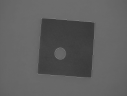the same two images as above were blended with X=0.7.

The bad result in the first example is mainly due to the low initial contrast in the input images. So, we will have a better result if the input images are of high contrast. To produce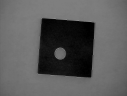the input images were contrast-enhanced with contrast stretching and then blended with X = 0.5. Although this already yields a better result, we still lose some contrast with respect to the original input images.

To maintain the full contrast in the output image we can define a special mask. The mask is made by thresholding the edge image at a pixel value of 128 and setting the non-zero values to one. Now, we blend the graylevel edge image (now corresponding to) and the original image using the thresholded image as a blending mask X(i,j). The imageshows the result, which is identical to the one achieved with image addition, but now achieved via a slightly simpler process.

Blending can also be used to achieve nice effects in photographs. We obtained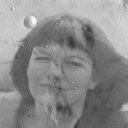by blending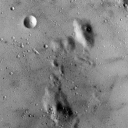with the resized version ofusing the X=0.5.

## Interactive Experimentation

You can interactively experiment with this operator by clicking here.

## Exercises

1. Examine the effects of using blending ratios other than 0.5 when blendingand2. Take an image and add a constant value (e.g. 100) using image blending and image addition. Comment on the differences of the results.
3. Produce a skeleton fromusing skeletonization. Assess the result by combining the two images using the blending operator.

## References

E. Davies Machine Vision: Theory, Algorithms and Practicalities, Academic Press, 1990, Chap. 2.

## Local Information